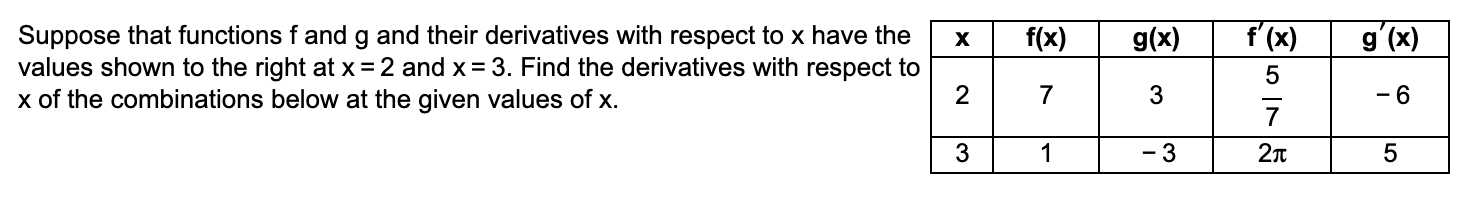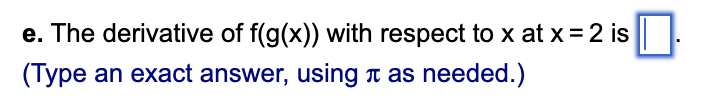# Question Solved1 AnswerХ f(x) g(x) g'(x) Suppose that functions f and g and their derivatives with respect to x have the values shown to the right at x = 2 and x = 3. Find the derivatives with respect to x of the combinations below at the given values of x. 2 7 3 f'(x) 5 7 2л vo -6 3 1 -3 5 e. The derivative of f(g(x)) with respect to x at x = 2 is (Type an exact answer, using a as needed.)Transcribed Image Text: Х f(x) g(x) g'(x) Suppose that functions f and g and their derivatives with respect to x have the values shown to the right at x = 2 and x = 3. Find the derivatives with respect to x of the combinations below at the given values of x. 2 7 3 f'(x) 5 7 2л vo -6 3 1 -3 5 e. The derivative of f(g(x)) with respect to x at x = 2 is (Type an exact answer, using a as needed.)
More
Transcribed Image Text: Х f(x) g(x) g'(x) Suppose that functions f and g and their derivatives with respect to x have the values shown to the right at x = 2 and x = 3. Find the derivatives with respect to x of the combinations below at the given values of x. 2 7 3 f'(x) 5 7 2л vo -6 3 1 -3 5 e. The derivative of f(g(x)) with respect to x at x = 2 is (Type an exact answer, using a as needed.)